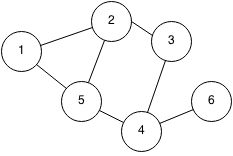## Introduction

A graph can be represented in various ways, but the most useful representations are as an adjacency matrix or as an adjacency list.

An adjacency matrix stores a graph of N nodes with a two dimensional NxN array. We let adjMatrix[i][j] represents the weight between the node i and node j. If we have a sparse graph which has many nodes but few edges, the memory storage will be very inefficient and it would be better to use an adjacency list. However, if the graph is very dense, or there are few nodes, then an adjacency matrix is quick and easy to use. Checking if an edge exists between two nodes is O(1).

Example:The adjacency matrix of the graph is:

1 2 3 4 5 6
1 0 1 0 0 1 0
2 1 0 1 0 1 0
3 0 1 0 1 0 0
4 0 0 1 0 1 1
5 1 1 0 1 0 0
6 0 0 0 1 0 0

An adjacency list stores a graph in an array of linked lists. Each node stores its neighbours by keeping a linked list of edges. Adjacency lists are slower when checking if an edge exists but they are more memory efficient since they only need to store the total number of edges.

Example:The adjacency list of the graph is:

Node edges
1 2 5
2 1 3 5
3 2 4
4 3 5 6
5 1 2 4
6 4